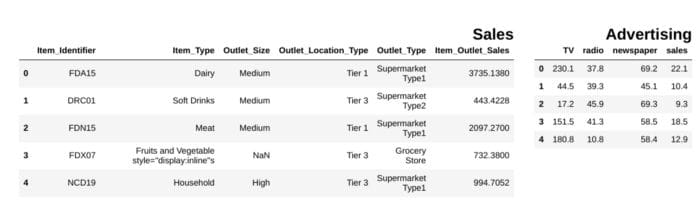By Lucas Soares, Machine Learning Engineer at K1 Digital

Photo by Carlos Muza on Unsplash

## Why Snippets Matter for Data Science

In my daily routine I have to deal with a lot of the same situations from loading csv files to visualizing data. So, to help streamline my process I created the habit of storing snippets of code that are helpful in different situations from loading csv files to visualizing data.

In this post I will share 15 snippets of code to help with different aspects of your data analysis pipeline

``````import glob
import pandas as pd
csv_files = glob.glob("path/to/folder/with/csvs/*.csv")
dfs = [pd.read_csv(filename) for filename in csv_files]``````

## 2. Getting unique values from a column table

``````import pandas as pd
df["Item_Identifier"].unique()array(['FDA15', 'DRC01', 'FDN15', ..., 'NCF55', 'NCW30', 'NCW05'],
dtype=object)``````

## 3. Display pandas dataframes side by side

``````from IPython.display import display_html
from itertools import chain,cycledef display_side_by_side(*args,titles=cycle([''])):
# source: https://stackoverflow.com/questions/38783027/jupyter-notebook-display-two-pandas-tables-side-by-side
html_str=""
for df,title in zip(args, chain(titles,cycle(['</br>'])) ):
html_str+='<th style="text-align:center"><td style="vertical-align:top">'
html_str+="<br>"
html_str+=f'<h2>{title}</h2>'
html_str+=df.to_html().replace('table','table style="display:inline"')
html_str+='</td></th>'
display_html(html_str,raw=True)
### Output``````image by the author

## 4. Remove all NaNs in pandas dataframe

``````df = pd.DataFrame(dict(a=[1,2,3,None]))
df
df.dropna(inplace=True)
df``````## 5. Show number of NaN entries in DataFrame columns

``````def findNaNCols(df):
for col in df:
print(f"Column: {col}")
num_NaNs = df[col].isnull().sum()
print(f"Number of NaNs: {num_NaNs}")
df = pd.DataFrame(dict(a=[1,2,3,None],b=[None,None,5,6]))
findNaNCols(df)# OutputColumn: a
Number of NaNs: 1
Column: b
Number of NaNs: 2``````

## 6. Transforming columns with `.apply` and lambda functions

``````df = pd.DataFrame(dict(a=[10,20,30,40,50]))
square = lambda x:...``````